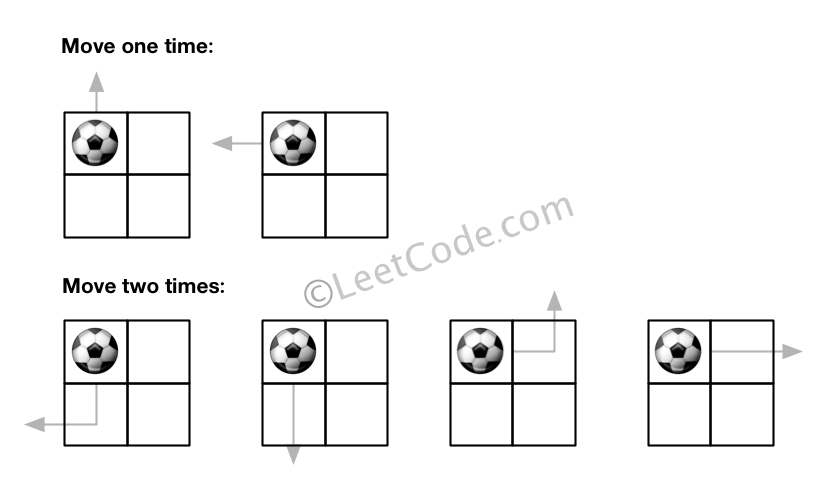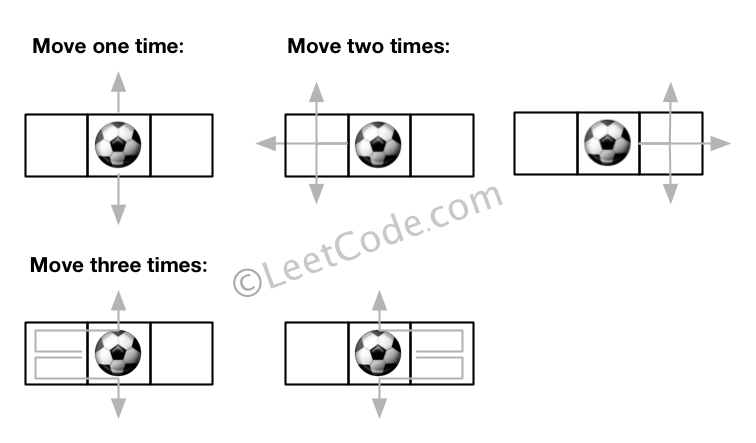## 576. Out of Boundary Paths

There is an m by n grid with a ball. Given the start coordinate (i,j) of the ball, you can move the ball to adjacent cell or cross the grid boundary in four directions (up, down, left, right). However, you can at most move N times. Find out the number of paths to move the ball out of grid boundary. The answer may be very large, return it after mod 109 + 7.

Example 1:

```Input: m = 2, n = 2, N = 2, i = 0, j = 0
Output: 6
Explanation:```

Example 2:

```Input: m = 1, n = 3, N = 3, i = 0, j = 1
Output: 12
Explanation:```

Note:

1. Once you move the ball out of boundary, you cannot move it back.
2. The length and height of the grid is in range [1,50].
3. N is in range [0,50].

## Rust Solution

``````struct Solution;
use std::collections::HashMap;

const MOD: i32 = 1_000_000_007;

impl Solution {
fn find_paths(m: i32, n: i32, k: i32, i: i32, j: i32) -> i32 {
let mut memo: HashMap<(usize, usize, usize), i32> = HashMap::new();
let m = m as usize;
let n = n as usize;
let k = k as usize;
let i = i as usize;
let j = j as usize;
Self::dp(i, j, k, &mut memo, m, n)
}
fn dp(
i: usize,
j: usize,
k: usize,
memo: &mut HashMap<(usize, usize, usize), i32>,
n: usize,
m: usize,
) -> i32 {
if k == 0 {
0
} else {
if let Some(&res) = memo.get(&(i, j, k)) {
return res;
}
let top = if i > 0 {
Self::dp(i - 1, j, k - 1, memo, n, m)
} else {
1
};
let left = if j > 0 {
Self::dp(i, j - 1, k - 1, memo, n, m)
} else {
1
};
let bottom = if i + 1 < n {
Self::dp(i + 1, j, k - 1, memo, n, m)
} else {
1
};
let right = if j + 1 < m {
Self::dp(i, j + 1, k - 1, memo, n, m)
} else {
1
};
let mut res = 0;
res += top;
res %= MOD;
res += left;
res %= MOD;
res += right;
res %= MOD;
res += bottom;
res %= MOD;
memo.insert((i, j, k), res);
res
}
}
}

#[test]
fn test() {
let m = 2;
let n = 2;
let k = 2;
let i = 0;
let j = 0;
let res = 6;
assert_eq!(Solution::find_paths(m, n, k, i, j), res);
let m = 1;
let n = 3;
let k = 3;
let i = 0;
let j = 1;
let res = 12;
assert_eq!(Solution::find_paths(m, n, k, i, j), res);
let m = 36;
let n = 5;
let k = 50;
let i = 15;
let j = 3;
let res = 390153306;
assert_eq!(Solution::find_paths(m, n, k, i, j), res);
}
``````

Having problems with this solution? Click here to submit an issue on github.